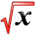# Math Differential EquationsThis article is part of the MathHelp Tutoring Wiki

• Q There is a patient whose blood drug level y (t ) you are trying to monitor. Suppose the drug is delivered intravenously at a rate of a per unit time and is lost from the kidney

at a rate of by( t) where a and b are positive constants.

A) Find the differential equation satisfied by y ( t ) .

B ) Suppose the blood drug level is 0 at t = 0. Find y(t ).

C) What does the blood drug level approach for large t?

D) What will happen to the blood drug level if the patient suddenly suffers from kidney dysfunction (that is to say, b becomes smaller)?

• A** A. first we form the differential equation y'(t) = a - by(t), or y' = a - by.

B. Then, we solve it by writing dy/dt = a - by. Rearrange the terms and integrate to get int dy/(a - by) = int dt --> -1/b ln|a - by| = t + C. Again, rearrange the terms to get a - by = De^{bt}, where I let D = e^{-bC} to save some typing. Plug in the initial condition (y = 0 when t = 0) to find D = a. Plug it in to get y(t) = a/b - a/b*e^{-bt}

C. as t --> ∞, y --> a/b

D. If kidney fails, then the differential equation becomes y' = a. Solving dy = adt yields y = at + C, where C is a constant. Initial condition of y = 0 when t = 0 tells us C = 0. Then y = at, and the person will soon die of having too much drug.